中文版 ENGLISH
 Home About SKLFS News and Events Research People Facilities Exchange and Cooperation Related Links Sitemap
 Wang XH (2001) Physical Review E 63(4), art. no.-047302. Date: 2011-08-15   Author: SKLFS  ,   Source: WOS  ,
 Wang XH (2001) Regular and anomalous scaring of a randomly advected passive scalar. Physical Review E 63(4), art. no.-047302. [In English] Web link: Keywords:hydrodynamic turbulence, conditional statistics, scaling functions, fusion rules, field,Abstract: Extending Kolmogorov's refined similarity hypothesis to study the inertial behavior [[T(x+r,t) -T(x,t)](2n)]proportional to r(zeta 2n) of a passive scalar T(x, t) advected by a rapidly changing incompressible velocity field, a random variable theta was introduced by Ching [Phys. Rev. Lett. 79, 3644 (1997)]. In this paper, the statistical distribution of the random variable X= theta/root[theta (2)] is investigated analytically for the scaling in two limits, n-independent scaling zeta (2n)=zeta (2) and regular scaling zeta (2n)=n zeta (2), and numerically for the scaling of the Kraichnan conjecture zeta (2n)=1/2 [root 4nd zeta (2)+(d-zeta (2))(2)- (d-zeta (2))]. For n-independent scaling zeta (2n) = zeta (2), the statistical distribution of X tends to an exponential distribution when zeta (2)-->,0 or d-->,infinity and to a Gaussian distribution when 52 -->,2 and d=2. For regular scaling zeta (2n)=n zeta (2), the statistical distribution of X tends to a Gaussian distribution when zeta (2)-->,0 or d-->,infinity. In d=2, there seems to be a phase transition for the probability density function P(X) from a convex to a concave function when the value of 52 is increased and the critical point is zeta (2)=4/3 where the random variable X has a uniform distribution in [-root3, root3]. In d=3, P(X) is a convex function for all 0<,<,zeta>,(2)<,2 and tends to a constant on its support [-<,root>,3, root3] when zeta (2)-->,2. For the scaling of the Kraichnan conjecture, P(X) has two peaks in d=2 for zeta (2)>,1.33, but, in d=3, it has only one peak for all 0<,<,zeta>,2<,2 and changes very slowly with the value of X in the neighborhood of X=O as <,zeta>,(2)-->,2.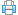Print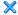Close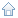State Key Laboratory of Fire Science, University of Science and Technology of China Jinzhai Road 96, Hefei, Anhui, P. R. China P. O.: 230026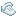Tel:(+86)551 63601651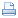Fax:(+86)551 63601669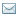E-mail:sklfs@ustc.edu.cn